# AP Chemistry : Thermochemistry and Kinetics

## Example Questions

### Example Question #1 : Kinetic Molecular Theory

Why does a higher temperature result in a faster reaction?

It increases the number of effective molecular collisions

It increases the surface area for reactions

It increases the pressure of the reactants

It helps orient reactants into the correct position to react

It lowers the activation energy for a reaction

It increases the number of effective molecular collisions

Explanation:

The temperature of a system can be manipulated to increase the reaction rate. The temperature affects the rate of the reaction because as temperature increases, so does the average kinetic energy. This means that molecules (reactants) are moving around more quickly, resulting in more frequent molecular collisions, resulting in an increased rate of product formation.

### Example Question #2 : Kinetic Molecular Theory

Which of the following statements in not an assumption made in kinetic molecular theory?

No attractive forces exist between gas molecules

The average kinetic energy of gas molecules is a function of both temperature and pressure

Collisions between gas molecules and the container are perfectly elastic

Much of the volume of the container is empty space

The average kinetic energy of gas molecules is a function of both temperature and pressure

Explanation:

The average kinetic energy is considered to be a sole function of temperature; pressure is not a factor. All of the other statements are assumptions of kinetic molecular theory.

### Example Question #3 : Kinetic Molecular Theory

The reaction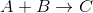obeys the rate law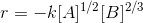What are the units of the rate constant,?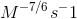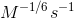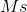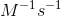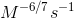Explanation:

Recall that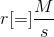and thatandare both molar concentrations. Then,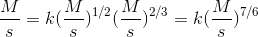Dividing both sides by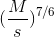gives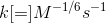### Example Question #4 : Kinetic Molecular Theory

Consider the following first order reaction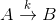Where k is the rate constant.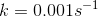and the initial concentrations of A and B are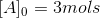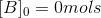What is the percent conversion after 500 seconds? Round to the nearest percent.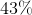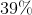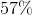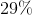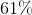Explanation:

For a first order reaction,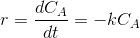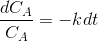Integrating both sides gives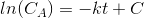Whereis an integration constant.

Solving for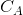gives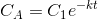Initially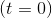there are 3 mols of. Using this we find that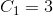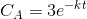At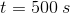,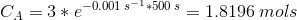Percent conversion is calculated as follows: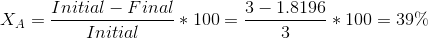### Example Question #1 : Reaction Rate And Rate Law

In a third order reaction with two reactants, if you triple the concentration of one of the reactants, the rate increases by a factor of 3. What happens to the rate of the reaction if the concentration of the second reactant is halved?

It doubles.

It decreases by a factor of 4.

It is halved.

It increases by a factor of 4.

It decreases by a factor of 4.

Explanation:

Based on the question, you can tell that the rate is first order with respect to the first reactant. So if the overall reaction is third-order, that means that the exponents must add to 3. We know that the exponent of the first reactant is 1, so that must mean that the exponent of the second reactant is 2. Thus, the concentration will be squared when you account for how it contributes to the rate, so the rate increases by a facotor of 4.

### Example Question #2 : Reaction Rate And Rate Law

Given the following. What is the rate law for the reaction A + 2B → C

[A](M)         [B] (M)          Rate (M/s)

0.1               0.02             0.005

0.2               0.02             0.02

0.1               0.01             0.0025

Rate  = k [A] [B]2

Rate = k [B]

Rate = k [A]

Rate = k [A] [B]

Rate = k [A]2[B]

Rate = k [A]2[B]

Explanation:

The rate is only based on the experimental values in the table. When B doubles and A stays the same the rate doubles, making it first order with respect to compound B. When compound A doubles and B stays the same, the rate quadruples, making it second order in regards to A.

### Example Question #3 : Reaction Rate And Rate Law

The rate of the reaction 2A + B → C was measured at different concentrations of reactants. The results are as follows:

[A]    [B]      Rate

0.5    2        0.1

0.5    4        0.4

1       2        0.2

r = k[A][B]

r = k[B]

r = k[A][B]2

r = k[A]2[B]

r = k[A]

r = k[A][B]2

Explanation:

When the concentration of A stays the same but the concentration of B doubles, the rate quadruples, showing a second order rate law based on B. When the concentration of A doubles and B stays the same, the rate also doubles, showing a first order rate law based on A.

### Example Question #4 : Reaction Rate And Rate Law

A rate law __________.

always requires experimental data to determine

can always be derived from looking at the reaction in question

can never be completely independent of the concentrations of any of the reactants

is measured in unit of concentration over time

is measured in unit of concentration over time

Explanation:

Rate laws can rarely be determined from just the reaction; they usually require experimental data. However, both of the answer choices contain the word ALWAYS, which is too extreme. Rate laws can be independent of the reactants, these rate laws are known as zero-order.

### Example Question #5 : Reaction Rate And Rate Law

Consider the following balanced equation.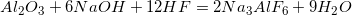The rate law for this reaction is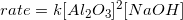.

What is the rate constant for this reaction if the reaction proceeds at an initial rate of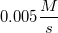when all of the reactants have initial concentrations of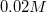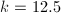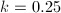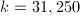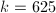Explanation:

Since the rate law is provided for the reaction, we can plug in the values that are relevant to the rate of the reaction. This will allow us to determine the rate constant.Note that HF is not included; the reaction must be zero order with respect to HF.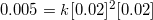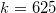### Example Question #6 : Reaction Rate And Rate Law

Consider the following reaction and experimental data.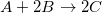Trial [A] (M) [B] (M) Initial reaction rate (M/s) 1 0.02 0.03 0.005 2 0.02 0.09 0.005 3 0.04 0.09 0.020

What is the rate law for this reaction?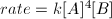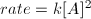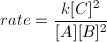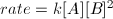Explanation:

When determining the rate law for a reaction, you need to determine the order for each reactant in the reaction. This can only be accomplished by performing an experiment where different trials show how the initial reaction rate changes based on the initial concentrations of the reactants. By keeping one initial reactant concentration constant and changing the concentration of the other reactant, we can see how the initial rate changes for each reactant.

For reactant A, we notice that the rate quadruples when its concentration is doubled by comparing trials 2 and 3. The concentration of A increases from 0.02 to 0.04, causing the rate to change from 0.005 to 0.020. This means that the order for reactant A is 2.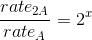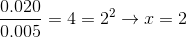For reactant B, we see that the rate does not change when the initial concentration of B is tripled by comparing trials 1 and 2. As a result, the reaction is 0 order wih respect to B.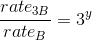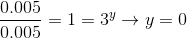Now that we have the orders for each reactant, we can write the rate law accordingly.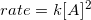Since anything to the 0 power is 1, B is omitted from the rate law and can be considered to be equal to 1.